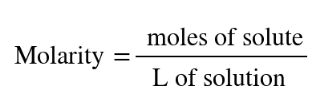# Problem: How many moles of solute particles are present in 1 mL of each of the following aqueous solutions?(d) 0.05 M (NH4)2CO3

###### FREE Expert Solution
• We can determine the moles of solute by using the provided volume and molarity where molarity is equal to:• Ionic molecule (NH4)2CO3, dissociates at water as:

(NH4)2CO(aq) → 2 NH4+ (aq) + CO32- (aq)###### Problem Details

How many moles of solute particles are present in 1 mL of each of the following aqueous solutions?

(d) 0.05 M (NH4)2CO3